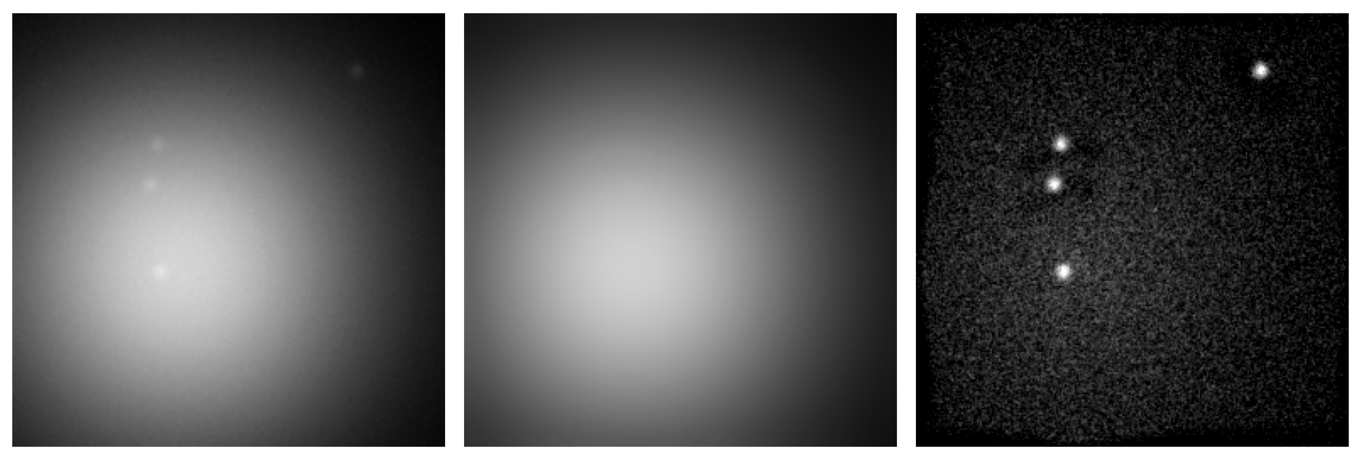## Prerequisites

Before starting this lesson, you should be familiar with:

## Learning Objectives

After completing this lesson, learners should be able to:
• Understand how to use image filters for creating a local background image

• Use the generated local background image to compute a foreground image

## Motivation

Very often, biological images contain locally varying background intensities. This hampers both segmentation and intensity quantification. However, often it is possible to generate a background image that can be subtracted in order to yield a foreground image with zero background. It is very important to know about this, because removing spatially varying background is a prevalent task in bioimage analysis.

## Concept map

graph TD ii(Input image) ii --> bgi[Background image] bgi --> s[Subtract] ii --> s s --> fgi[Foreground image]

## FigureLocal background correction using a median filter. Left - Raw data. Middle - Median filtered image (background). Right - Difference image (foreground).

## Activities

#### Background subtraction using a median filter

• Open image xy_8bit__some_spots_with_uneven_bg
• Compute a background image using a median filter
• Create a foreground image by subtracting the background image from the input image
• (Optional) Segment the spots in the foreground image.

Show activity for:

## ImageJ GUI

• Open image xy_8bit__some_spots_with_uneven_bg
• [ Image › Rename… ]
• “input”
• Create background image
• [ Image > Duplicate…]
• [ Process > Filters > Median… ]
• [ Image › Rename… ]
• “background”
• Create foreground image
• [ Process › Image Calculator… ]
• Image 1 = input
• Subtract
• Image 2 = background
• create
• 32-bit
• [ Image › Rename… ]
• “foreground”

## skimage napari

#### Background subtraction using a maximum intensity projection

• Open image xyt_8bit_polyp
• Create a maximum intensity projection of this image.
• Because the polyp is moving around and is darker than the background this will create a background image.
• Create a foreground image by subtracting the maximum intensity projection from the original image.

Show activity for:

## ImageJ GUI

• Open image xyt_8bit_polyp
• Make a maximum intensity projection to create a background image ([Image › Stacks › Z Project…])
• Use the image calculator function [ Process › Image Calculator…] to subtract the maximum intensity projection from the original:
• Image1: xyt_8bit_polyp
• Operation: Subtract
• Image2: MAX_xyt_8bit_polyp
• ‘create new window’
• ‘32-bit float result’
• Say ‘yes’ to the ‘Process entire stack’ message.

## Assessment

### True or false?

1. Mean filter is better than the median filter to generate a background image.
2. On the generated background image the objects of interest should not be visible.
3. When creating a background image by means of filtering: The size of the filter’s structuring element should be much smaller than the size of the objects.

## Solution

1. False (mean filter is really quite poor in terms of removing foreground information)
2. True (because this is the background image, so it should not contain any foreground information)
3. False (it should be much (maybe ~3 times) larger in order to remove the objects from the image)

## Explanations

There exist multiple methods on how to compute a background image. Which methods and parameters work best depends on the specific input image and the size of the object of interest.

Common methods are:

• Median filtering
• Morphological opening. Subtraction of the opened image from the original image is also called Top-Hat filtering.
• Rolling ball, this alogorithm is implemented for instance in ImageJ `Background Subtraction` or `skimage.restoration.rolling_ball`

Some of the methods may be sensistive to noise. Therefore, it can be convenient to smooth the image, e.g. with a mean or gaussian filtering, prior computing the background image.

## Follow-up material

Recommended follow-up modules: